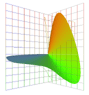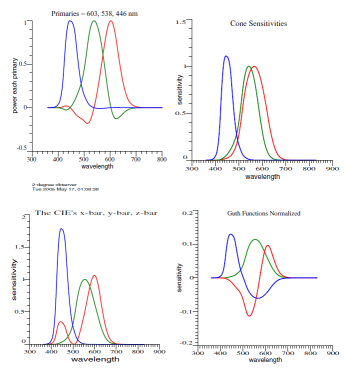Jim Worthey • Lighting & Color Research • jim@jimworthey.com • 301-977-3551 • 11 Rye Court, Gaithersburg, MD 20878-1901, USA

Question: What is the proof that Jozef Cohen’s Matrix R is invariant?

Proof that Projection Matrix R = A(A'A)−1A' is Invariant

To begin one may ask "With respect to what is R invariant?" Consider this miniature version of Fig. 1 in the Vectorial Color manuscript (or article):This is a mini version of Fig. 1 in the Vectorial Color manuscript. At upper left is a set of color-matching data as they might appear an experiment, and next comes human cone sensitivities. Then the familiar x-bar, y-bar, and z-bar are displayed, and the last graph shows a set of opponent-color functions.

The four graphs look dissimilar, but are related. If they are used as color-matching functions, all 4 sets of functions predict the same color matches. The fictitious experimental data, the cone sensitivities, and the opponent functions were all computed by adding and subtracting the CIE's three functions. Each set of functions is a linear combination of any other set.

In short, the facts of color matching have alternate representations. That is not a new idea, but part of our legacy of well-known color science from the 19th and 20th centuries. Cohen discovered something new, that projection Matrix R comes out the same, no matter which set of color matching functions is chosen as a starting point1.

Matrix Theorems: We'll need a couple theorems about matrices. The prime symbol, ', denotes matrix transpose.

• Transpose of a matrix product:   (AB)' = B' A'  .         (1)
• Inverse of a matrix product:    (AB)−1B−1 A−1   .      (2)
• Extending the idea of Eq. (2):     (ABC)−1C−1 B−1 A−1   .      (3)
In Eq. (2) and (3), matrices A, B, C must be square and the inverses must exist. In Eq. (1), A and B need not be square, but must be conformable for multiplication.

Invariance of R: Now suppose that the columns of A are a set of color matching functions, such as the cone sensitivities. Let X be an invertible square matrix that transforms A to a different set of CMFs.
• "Old" cmfs:  A
• "New" cmfs: AX
If A is the given set of CMFs, then
R = A(A'A)−1A'      .             (4)

If the CMFs are AX, then
R = AX[(AX)'(AX)]−1(AX)'      .             (5)

R = AX[X'A'AX]−1X'A'      .             (6)
Apply Eq. (3) :
R = AXX−1(A'A)−1X'−1X'A'      .             (7)

R = A(A'A)−1A'      .             (8)

Eq. (8) is the same as Eq. (4), therefore R is invariant.

1. Jozef B. Cohen and William E. Kappauf, “Metameric color stimuli, fundamental metamers, and Wyszecki’s metameric blacks,” Am. J. Psych. 95(4):537-564 (1982).